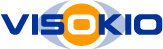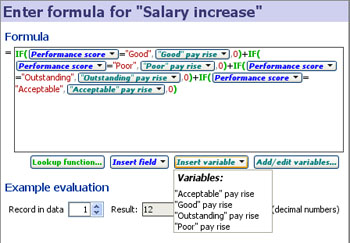# Defining and Editing Formulae

### Using the interactive, visual Formula Editor

The Formula Editor dialog in Omniscope is a powerful tool for creating and editing an Omniscope formula. For more general information about adding formulae to your files, see Formula Fields.## The formula text box

The large white box is the formula itself.  This box can be edited just like a text field in a form.  You can type your formula in from scratch, or use the buttons underneath to help you insert elements of the formula.

#### References

Field and variable references are shown inside the text as little embedded buttons, but these behave like characters in the text, and can be selected, cut, copied, pasted and deleted just like other text. Additionally, you can click each embedded button to change the field or variable.

Field references can be inserted using the Insert field below the formula box.  Variable references can be inserted using Insert variable except you will need to define the variable beforehand using

Alternatively, you can type the field/variable name directly (but if it has punctuation or spaces, you will need to enclose the name in [square brackets]).

#### Syntax highlighting

Colours are used to identify different parts of the formula.  For example, green indicates a function such as SUM(...), blue indicates a field reference, and cyan/turquoise indicates a variable reference.

#### Undo/redo

During a session with the formula editor, use Ctrl+Z to undo your edits, and Ctrl+Y or Ctrl+Shift+Z to redo edits you have undone.  Accidentally deleted half an hour's work? Ctrl+Z will bring it back.

Once you have clicked OK, the edited formula will be applied and your fields will recalculate.  At this point, from the main Omniscope window, you can use Data > Undo edit or press Ctrl+Z to undo the entire formula editing session (from the point you opened the formula editor until you pressed OK).

#### Inserting functions

If you know the function, simply type its name followed by an open bracket, e.g. "SUM(".  Then provide any arguments/parameters to the function, separated by commas, ending with a close bracket.  For example: "SUM(1, 2)".

If you are unsure how to use a particular function, type the function name and the open bracket, then move your mouse over the function.  A tooltip will appear with guidance.

To browse and insert functions from a list, click Functions list.  In the dialog that appears, browse through the functions and read their syntax.  Find the function you want and click OK.  The function and sample usage will be inserted into the formula at the cursor position.  This will typically introduce errors which will be highlighted, since you must change the sample argument/parameter names for real values, references or expressions.  More...

All standard spreadsheet functions are provided where appropriate for Omniscope, so you can expect POWER() to work in the same way as Excel, for example.  Additional Omniscope-specific functions such as SUBSET are also provided.

See the Knowledge Base Functions Guide for full documentation of all functions.

Long and complicated formulae can be hard to read and work with.  Use spaces, tabs, line-breaks and comments to space-out and describe sections of the formula, without affecting calculation.

Comments must be surrounded with "/*" and "*/" as in the following example:

(1 + 1) /* adding some numbers */

/ 2   /* dividing in half */

The formula editor window can also be resized as large as needed when working on a very large formula.

## Seeing the results

While editing your formula, you can see the results in two ways:

#### Previewing the result of the whole formula

In the section Example evaluation, use the Record in data spinner to select a record.  Providing your formula has no errors, the result will be shown to the right, along with the data type.  If you move the formula editor to one side, the same record will be highlighted in the views.

#### Previewing the result of parts of your formula

Move your mouse over the formula. As you move, different parts will become highlighted, and if you hold the mouse over a particular part, a tooltip will appear containing the result of that part of the formula when evaluated against the record chosen in Example evaluation

For example, in the formula "(1 + 1) * 2", moving your mouse over the "+" will highlight "1 + 1" and the tooltip will show "1 + 1 = 2". Moving over the "*" will highlight "(1 + 1) * 2", and the tooltip will show "(1 + 1) * 2 = 4".

## Errors

If your formula contains errors, these will be reported in red text in the Errors section.  Guidance text deemed useful for a function in the formula will be shown.  The relevant part of the formula will be highlighted in pink.

#### Common errors:

• Incomplete expression - for example, "1 + 2 +" is incomplete, because it lacks the last value.  Also, "(1+1 / 2" is missing a close bracket.
• Function "..." has the wrong number of parameters - for example, "SUM()" is wrong because SUM requires at least 1 parameter.  The usage guide for the function will be shown.
• Function parameters must be numeric - you are using a non-numeric value as a parameter to a function which requires numeric parameters.
• No such field or variable "..." - You have entered a reference that does not exist.  Use the Insert field button to insert field references, rather than typing them.  Or use Add/edit variables then Insert variable to insert variable references.  If you are trying to insert a function, you have forgotten to add open/close brackets after the name.